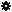﻿ Object Model: VertexCollection.GrowNeighborVertices

VertexCollection.GrowNeighborVertices

## VertexCollection.GrowNeighborVerticesv3.0

### Description

ReturnS a collection of Vertex objects for all vertices that are adjacent to each Facet of each Vertex in this collection, within the specified distance.

### C# Syntax

 ```VertexCollection VertexCollection.GrowNeighborVertices( Int32 in_lDistance ); ```

### Scripting Syntax

 ```oReturn = VertexCollection.GrowNeighborVertices( [Distance] ); ```

### Parameters

Parameter Type Description
Distance Integer Distance is an integer value representing the degree of neighborhood (eg: degree=2 for a vertex means its adjacent vertices plus the adjacent vertices of the adjacent vertices)

Default Value: 1

### Examples

#### VBScript Example

 ```set oCube = ActiveSceneRoot.AddGeometry( "Cube", "MeshSurface" ) set oGeometry = oCube.ActivePrimitive.Geometry set oVertices = oGeometry.Polygons(0).Vertices set oNeighborVertices = oVertices.GrowNeighborVertices str = "Vertices around the vertices of the polygon(0) are :" for each n in oNeighborVertices str = str & " " & n.index next logmessage str ```